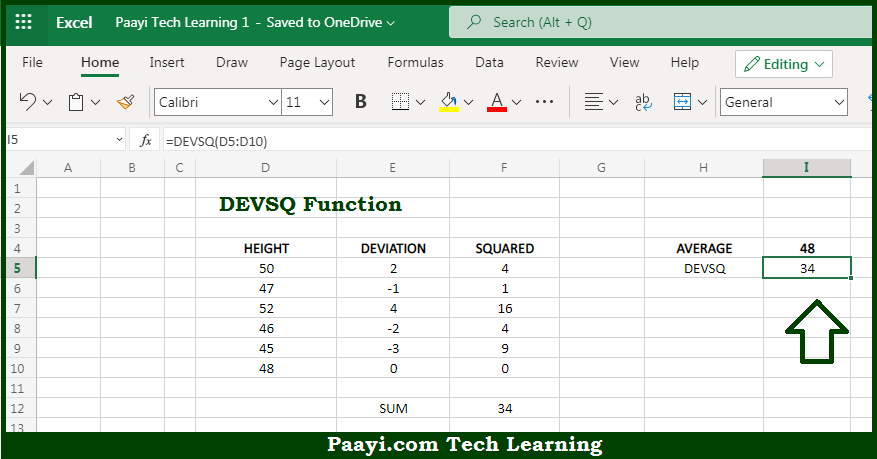# Learn How to Use Microsoft Excel DEVSQ Function

Written by | 0 Comments | 536 Views

In this article, you will learn how to use the Microsoft Excel DEVSQ function and its prime function in Microsoft Excel. You will also get to know the Microsoft Excel DEVSQ function return value and syntax with the help of some examples.

Microsoft Excel DEVSQ Function

The main purpose of the Microsoft Excel DEVSQ function is to get the sum of squared deviations. That implies, with the help of the DEVSQ function you can able to return the sum of the squared deviations from the mean for a given set of data. It should be noted that the arguments can be numbers, names, arrays, or references that contain numbers and empty cells, and cells that contain text or logical values are ignored. So, with the help of the DEVSQ function, you can able to get the sum of squared deviations.

Return Value of DEVSQ Function

The return value will be the calculated sum.

Syntax of DEVSQ Function

=DEVSQ(number1, [number2], ...)

Where the arguments:

• number1: This is the first value or reference.
• number2: This is the second value or reference (optional).

## How to Use Microsoft Excel DEVSQ Function?So we know that Microsoft Excel DEVSQ function you can able to get the sum of squared deviations. That implies, with the help of the DEVSQ function you can able to return the sum of the squared deviations from the mean for a given set of data. It should be noted that the arguments can be numbers, names, arrays, or references that contain numbers and empty cells, and cells that contain text or logical values are ignored. So, with the help of the DEVSQ function, you can able to get the sum of squared deviations.